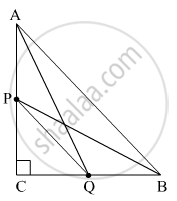# If P and Q Are the Points on Side Ca and Cb Respectively of δAbc, Right Angled at C, Prove that (Aq2 + Bp2 ) = (Ab2 + Pq2) - Mathematics

Sum

If P and Q are the points on side CA and CB respectively of ΔABC, right angled at C, prove that (AQ2 + BP2 ) = (AB2 + PQ2)

#### SolutionUsing the Pythagoras theorem in ΔABC, ΔACQ, ΔBPC, ΔPCQ, we get
AB2 = AC2 + BC2                  ......(1)
AQ2 = AC2 + CQ                ......(2)
BP2 = PC2 + BC                  .......(3)
PQ2 = PC2 + CQ                .......(4)

Adding the equations (2) and (3) we get
AQ2 + BP2 = AC2 + CQ2 + PC2 + BC2
= (AC2 + BC2) + (CQ + PC2)
= AB2 + PQ2

As
L.H.S. = AQ2 + BP2
= AB2 + PQ2
= R.H.S
Hence Proved

Concept: Right-angled Triangles and Pythagoras Property
Is there an error in this question or solution?